## Table of ContentOpen Access

ARTICLE

# An Improved Bald Eagle Search Algorithm with Cauchy Mutation and Adaptive Weight Factor for Engineering Optimization

Wenchuan Wang1,*, Weican Tian1, Kwok-wing Chau2, Yiming Xue1, Lei Xu3, Hongfei Zang1

1 College of Water Resources, Henan Key Laboratory of Water Resources Conservation and Intensive Utilization in the Yellow River Basin, North China University of Water Resources and Electric Power, Zhengzhou, 450046, China
2 Department of Civil and Environmental Engineering, The Hong Kong Polytechnic University, Hong Kong, China
3 College of Hydrology and Water Resources, Hohai University, Nanjing, 210024, China

* Corresponding Authors: Wenchuan Wang. Email:;(This article belongs to this Special Issue: Computational Intelligent Systems for Solving Complex Engineering Problems: Principles and Applications)

Computer Modeling in Engineering & Sciences 2023, 136(2), 1603-1642. https://doi.org/10.32604/cmes.2023.026231

## Abstract

The Bald Eagle Search algorithm (BES) is an emerging meta-heuristic algorithm. The algorithm simulates the hunting behavior of eagles, and obtains an optimal solution through three stages, namely selection stage, search stage and swooping stage. However, BES tends to drop-in local optimization and the maximum value of search space needs to be improved. To fill this research gap, we propose an improved bald eagle algorithm (CABES) that integrates Cauchy mutation and adaptive optimization to improve the performance of BES from local optima. Firstly, CABES introduces the Cauchy mutation strategy to adjust the step size of the selection stage, to select a better search range. Secondly, in the search stage, CABES updates the search position update formula by an adaptive weight factor to further promote the local optimization capability of BES. To verify the performance of CABES, the benchmark function of CEC2017 is used to simulate the algorithm. The findings of the tests are compared to those of the Particle Swarm Optimization algorithm (PSO), Whale Optimization Algorithm (WOA) and Archimedes Algorithm (AOA). The experimental results show that CABES can provide good exploration and development capabilities, and it has strong competitiveness in testing algorithms. Finally, CABES is applied to four constrained engineering problems and a groundwater engineering model, which further verifies the effectiveness and efficiency of CABES in practical engineering problems.

## Keywords

1  Introduction

Metaheuristic algorithms have the advantages of convenient calculation, high accuracy and reliability. In several disciplines, they’ve been frequently employed in engineering optimization issues, such as physics, water conservancy, machinery, and structures. Therefore, meta-heuristic algorithms have become the best choice for solving complex engineering problems .

Depending on where they come from, metaheuristic algorithms may be categorized into four types, namely swarm-based, physics-based, human-based and evolutionary-based algorithms . Swarm-based algorithms involve a swarm of solutions in which each individual compares to each other to generate better solutions. Ant colonies, bird swarms, and fish swarms are examples of swarm intelligence algorithms, such as the Artificial Bee Colony algorithm (ABC) , Spider Monkey Optimization algorithm (SMO) , Particle Swarm Optimization algorithm (PSO) , Bald Eagle Search (BES) , etc. Physics-based algorithms imitate physical rules of the world, such as the Simulated Annealing algorithm (SA) , Archimedes Optimization Algorithm(AOA) , Multiverse Optimization algorithm (MVO) , Gravity Search Algorithm (GSA) , etc. The core idea of human based algorithm is to simulate a human behavior process, such as Human Learning Optimization algorithm (HLO) , Seeker Optimization Algorithm (SOA) , etc. Evolution based algorithms, inspired by biological evolution, have strong robustness. Evolution-based algorithms include Genetic Algorithm (GA) , Cooperative Co-Evolution Algorithm (CCEAs) , Estimation of Distribution Algorithm (EDA) , etc.

Among them, BES algorithm  has the advantages of simple initialization conditions and strong search capability, which can solve optimization problems well. In the past few years, BES has been used to solve a variety of real-world problems, e.g., designing the dispatching range, improving the overall efficiency of power system , predicting ozone concentration , forecasting traffic flow , finding optimal value of super parameters , improving the diagnosis accuracy of transformer winding fault , etc.

Although the BES algorithm has certain advantages, “there is no such thing as a free lunch” . BES algorithm still has some common problems of meta-heuristic algorithms. In solving practical problems, inaccurate location updates in the selection and search stages lead to local optimization in the process of searching complex functions. For this kind of problem, hybrid strategies have been employed to improve algorithms . Among them, the Cauchy mutation strategy and adaptive weight strategy significantly improve optimization algorithms.

Based on the results of the two strategies mentioned above [18,24,29,30], this paper proposes a bald eagle search algorithm (CABES) based on Cauchy mutation and adaptive weight optimization. Firstly, in the selection stage, the Cauchy mutation approach is used to increase global search optimization by boosting the search neighborhood’s local search capability. Secondly, an adaptive weight factor is used in the search stage to increase the solution space’s local search capabilities. Thirdly, the performance of CABES and other advanced swarm intelligence algorithms is evaluated qualitatively and quantitatively using the CEC2017 test set’s twenty-nine benchmark test functions and verifying the competitiveness of CABES in various algorithms . The testing and application of CABES in four practical engineering examples and a groundwater model show that it can effectively solve real-world constrained optimization problems. The following contributions are made by the proposed work:

(a)   Use the Cauchy mutation strategy to increase the search step size, and boost the CABES algorithm’s global exploration capability and the likelihood of discovering the global optimum.

(b)   An adaptive weight technique is presented to increase algorithm development accuracy and local search efficiency.

Below is a list of the remaining sections in this paper. The principle of the BES algorithm, the Cauchy mutation strategy and the adaptive weighting strategy are introduced in Section 2. In Section 3, CABES is explored. Section 4 compares the performance of the CABES algorithm to that of other algorithms using the CEC2017 test functions. The performance of CABES on real-world optimization issues is given in Section 5. The conclusion is made in Section 6.

2  Preliminary

2.1 BES Algorithm

The BES algorithm is a new meta-heuristic algorithm proposed by scholar Alsattar et al. . The bald eagle is widely distributed in North America, with keen vision and excellent observation ability in flight.

In the case of salmon prey, the bald eagle will first choose a search space based on the density of salmon individuals and populations and then search the water surface inside that search region. Finally, the bald eagle would lower its flight height gradually and plunge down rapidly to seize the prey. The mathematical model of each stage is as follows:

A.   selecting a search space:

To assist the search, the bald eagle chooses a search region at random and finds the optimal search location by assessing the amount of preys. In this stage, the bald eagle position update is determined by multiplying the preceding information from the random search, and the mathematical model is defined as Eq. (1):

Li,new=Lbest+pq(LmeanLi)(1)

where p is the control position variation parameter in the range (1.5, 2); the random number q ranges from 0 to 1; the optimal position for searching for bald eagles is Lbest; Lmean is the average distribution of bald eagle positions after the preceding search; Li corresponds to the i-th bald eagle.

B.   searching the space for prey (exploration phase):

The bald eagle searches for prey in a spiral pattern in the designated search zone, speeding up the search process to obtain the optimal dive catch position. The mathematical model of spiral flight, which adopts polar coordinate equation to update the position, is expressed as Eqs. (2) to (5):

θ(i)=spiq(2)

d(i)=θ(i)+tq(3)

dx(i)=d(i)sin(θ(i)),dy(i)=d(i)cos(θ(i))(4)

x(i)=dx(i)/max(|dx|),y(i)=dy(i)/max(|dy|)(5)

where θ(i) and d(i) are the spiral equation’s polar angle and diameter, respectively; s and t represent the spiral trajectory’s control parameters, the ranges of variation are (5, 10) and (0.5, 2), respectively; x(i) and y(i) are the vulture’s polar coordinates positions, and the values are (−1, 1). The bald eagle location is updated as Eq. (6):

Li,new=Li+x(i)(LiLmean)+y(i)(LiLi+1)(6)

where Li+1 is the i-th bald eagle’s next update position.

C.   swooping to capture the prey (utilization stage):

A bald eagle will fly quickly to its prey from a position determined in the previous phase, while others in the population will travel to their best positions and attack the prey simultaneously, and the motion is described by polar equations as Eqs. (7) to (10):

θ(i)=spiq,d(i)=θ(i)(7)

dx(i)=d(i)sinh(θ(i)),dy(i)=d(i)cosh(θ(i))(8)

x1(i)=dx(i)/max(|dx|),y1(i)=dy(i)/max(|dy|)(9)

The updating formula of the bald eagle position in the dive is:

Li,new=qLbest+x1(i)(Lic1Lmean)+y1(i)(Lic2Lbest)(10)

where c1 and c2 are the intensities of motion of the bald eagle toward the optimal and central positions, respectively, with values in the range of (1, 2).

2.2 Cauchy Mutation Strategy

The cauchy distribution is a unique distribution , which has long tail wings at both ends. The distribution feature gives individuals a higher probability of jumping to a better position and breaking away from local optimization. The peak value distributed at the center 0 is small, the trend from peak value to 0 is smooth, and the variation range is uniform.

One can express the probability density function of a one-dimensional Cauchy distribution as Eqs. (11) and (12):

f(x,η,μ)=1πηη2+(xμ)2,<x<(11)

when η=1,μ=0, the probability density function will become the standard form:

f(x,η,μ)=1π1x2+1,<x<(12)

The density function curves of the standard Cauchy distribution and the standard normal distribution are shown in Fig. 1. Liu et al.  carried out Cauchy mutation on the ant colony algorithm, as Eq. (13):

xi=xi(1+C(λ))(13)

where C is a random number in the Cauchy distribution, and xi represents the best current ant colony location.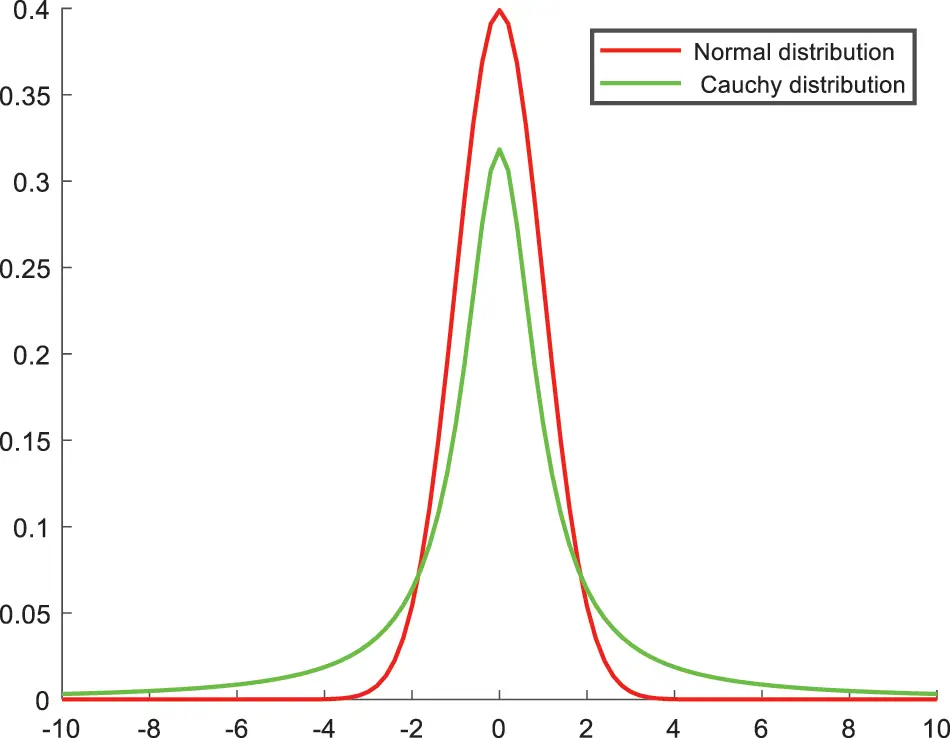Figure 1: Plots of cauchy distribution and standard normal distribution

The adaptive weighting factor is a very important parameter. Adding appropriate weight factors is helpful in improving the convergence accuracy and speed of the algorithm. For example, Gao et al. introduced the weight factor into the SSA algorithm to improve search accuracy .

Large scale global exploration is needed in the early stage of the algorithm, and small local development is needed in the late stage to avoid premature convergence of the algorithm. At the beginning of the iteration, the adaptive weight factor is large, which can make the algorithm search globally and help to find the optimal position. As the iteration continues, the algorithm may fall into local optima. At this time, the weight factor becomes smaller, which helps the algorithm to conduct local search, find the best solution finely, and jump out of the local optimum.

The adaptive weighting factor is shown in Eq. (14).

ω=sin(πit2Maxit+π)+1(14)

where Maxit is the maximum number of iterations, it is the number of iterations currently in progress.

3  CABES Algorithm

Algorithm description:

The main principle of BES is to imitate the three stages of eagle hunting. In the meta-heuristic intelligent optimization algorithm, the algorithm’s local and global search capabilities may be utilized to evaluate the optimization impact. In this paper, we improve the optimization mechanism for the first two stages of BES.

3.1 Improvement of Cauchy Mutation Strategy

In the search space selection stage, the bald eagle uses the available information in the previous stage to determine the next search area. If the eagle population falls into an optimal local state, it will not capture its prey accurately. It means that the algorithm will be unable to find the best solution to the optimization issue, thus reducing the optimization effect of the algorithm. Therefore, it is necessary to promote the global search ability of the bald eagle, as well as the optimization ability of the BES. The specific improvements are as follows:

The Cauchy mutation technique is used to broaden the population’s search area, allowing the BES algorithm to break free from the local optimum. Eq. (1) is changed to Eq. (15):

Li,new=Lbest+C(λ)(LmeanLi)(15)

where Li,new is the new position generated after introducing Cauchy improvement. Refer to the previous text for other parameters. Fig. 2 shows the BES pseudocode combined with Cauchy mutation.Figure 2: Pseudocode of cauchy mutation

In order to clearly observe the optimization process of the algorithm, four minimization functions (selected from the multimodal functions fun-6, fun-9, hybrid function fun-17 and composition function fun-21 in the CEC2017 test set) are used to draw the convergence process curve of the BES with Cauchy mutation and the original BES algorithm (Fig. 3).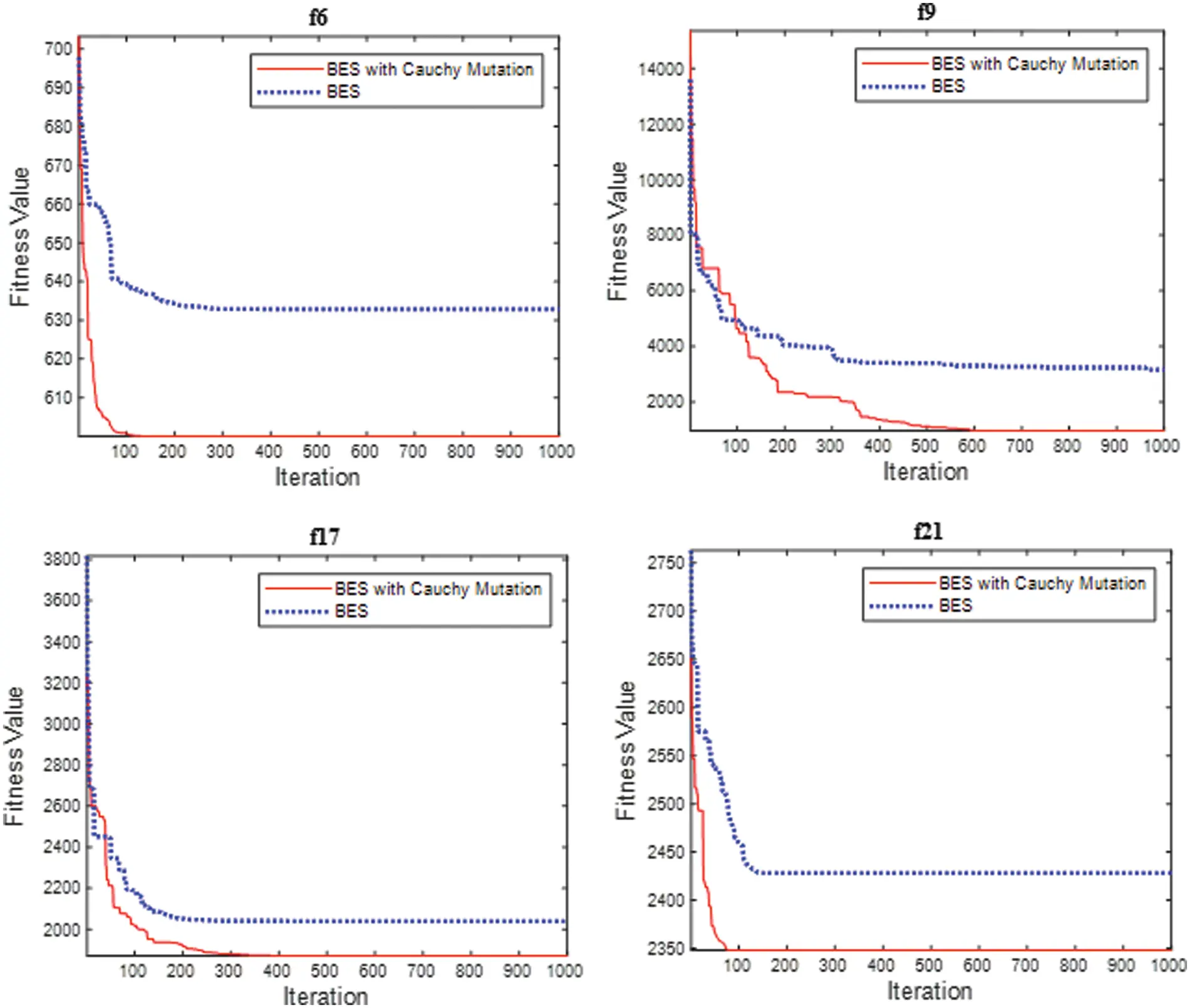Figure 3: BES iteration curve combined with cauchy mutation strategy

The results suggest that incorporating the Cauchy mutation approach into the algorithm may significantly increase the algorithm’s ability to discover optimal value information, and the optimization accuracy is also greatly improved. Cauchy mutation strategy is an effective improvement strategy.

3.2 Improvement of Adaptive Weight Strategy

In the BES algorithm, once the bald eagle has selected a search space, it begins to enter the prey search phase. However, the search phase of the algorithm only updates the location of the current population, ignoring the location information generated by other iterations of the algorithm. As a result of this, the algorithm lacks information when searching for and updating location, resulting in inaccurate location update and slow convergence speed.

Some academics use adaptive weight into other optimization algorithms in order to boost the algorithm’s capacity to optimize . To increase the algorithm’s local mining capacity, it is important to re-update the neighborhood of the search prey position, improve the original algorithm’s solution accuracy in the local neighborhood, and locate the best solution in the search space. After its introduction, Eq. (6) is changed to Eq. (16):

Li,new=ωLi,new(16)

By introducing the adaptive weight factor, the search location update formula is updated to make the update location more accurate and improve the local optimization capability of the bald eagle.

Fig. 4 shows the BES pseudocode with adaptive weight.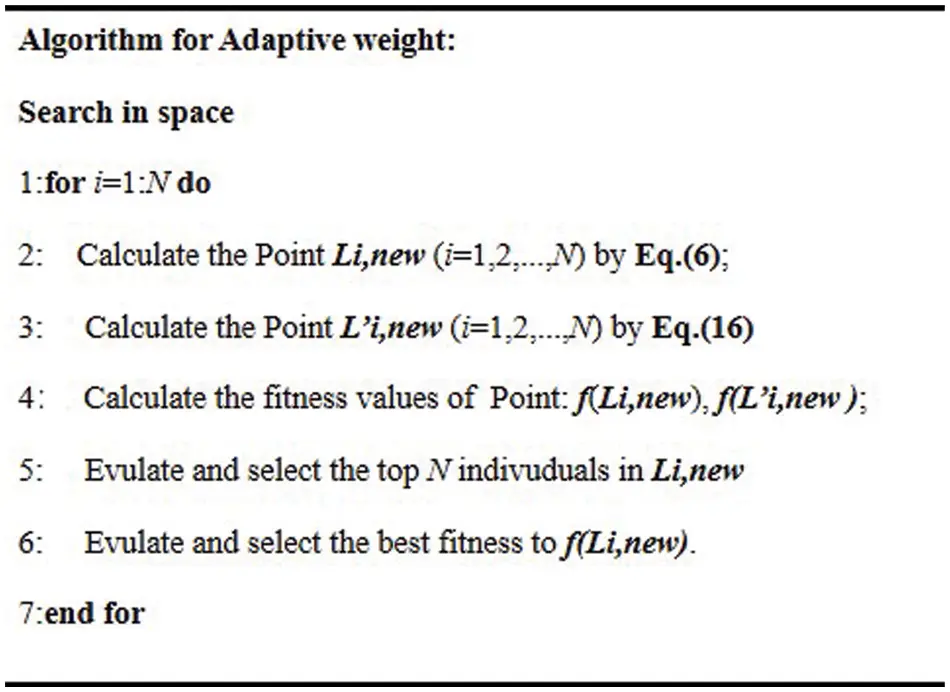Figure 4: Pseudocode of the adaptive weight strategy

In order to confirm the effectiveness of the adaptive weight strategy, four minimization functions (selected from the CEC2017 test set of functions fun-5, fun-8, fun-16, fun-23) are used to compare the BES algorithm improved by adaptive weight factor with the original BES algorithm.

Fig. 5 depicts the BES algorithm change curve after the adaptive weight factor was included. It has been discovered that adding the adaptive factor to the algorithm can assist it in getting rid of the local optimum and locating the global optimum. The effectiveness of the adaptive weight strategy is proved to some extent.Figure 5: BES iteration curve combined with the adaptive weight strategy

3.3 Combination of Different Improvement Strategies

Combining the above two improved strategies to promote the optimization capability of the bald eagle algorithm is named the bald eagle algorithm based on Cauchy and adaptive weight (CABES). Fig. 6 shows its pseudocode.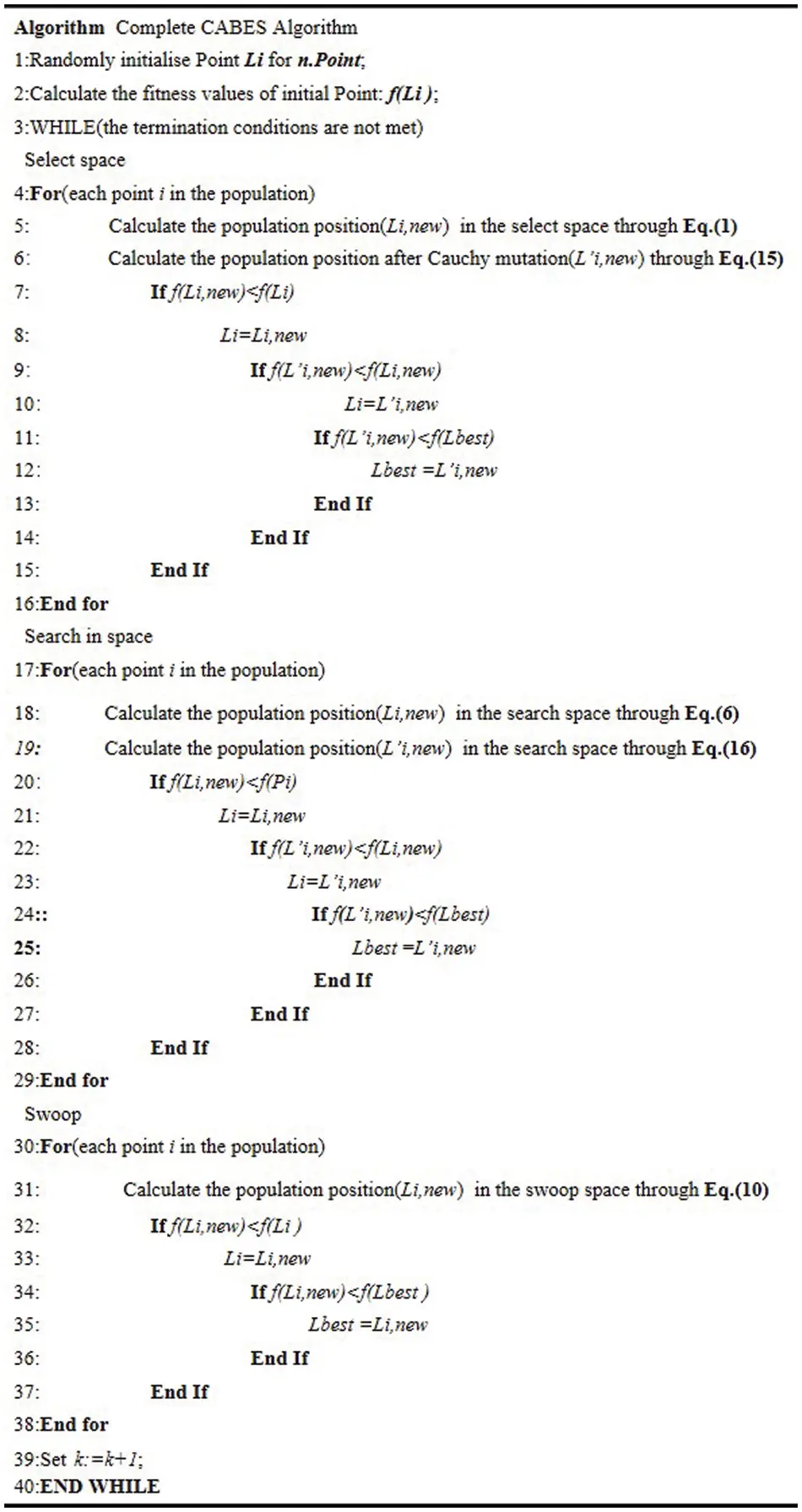Figure 6: Pseudocode of CABES

3.4 Time Complexity Analysis of CABES

It is assumed that N is the overall scale of the condor algorithm. The dimension of the objective function is D. The calculation time of the objective function is F. T is the maximum number of iterations; TC is the time complexity of the objective function.

In the original BES algorithm, it is computationally complex for the initial stage to be O(N), computing the initial population fitness is computationally complex in O (N * F * D), and the total time complexity of the three stages of selection, search and capture is O [(N * F + N * F + N * F) * T * D].

Total complexity:

TCBES = O(N) + O(N * F * D) + O[(N * F + N * F + N * F) * T * D]

Since the bald eagle algorithm mainly consumes time on the evaluation objective function, the time complexity of the original bald eagle algorithm can be approximated as:

TCBES = O [(N * F + N * F + N * F) * T * D] = O(3 * N * F * T * D)

In CABES, the operation of initialization parameters and computing population fitness is the same as that of BES, so the time complexity of CABES in the initialization phase is the same as that of BES, which is also O (N), and the complexity of computing initial population fitness is O (N * F * D). In the loop part of the algorithm, CABES introduces Cauchy mutation and adaptive operation respectively in the selection and search stages of BES, so it takes two more times to evaluate the objective function. As such, the total time complexity in the three stages of selection, search and capture is O[(N * F + N * F + N * F + N * F + N * F) * T * D].

Total complexity: TCCABES = O(N) + O(N * F * D) + O((N * F + N * F + N * F + N * F + N * F) * T * D]

Similarly, CABES mainly spends time evaluating the objective function, so the time complexity of the improved Condor algorithm is approximately:

TCCABES = O[(N * F + N * F + N * F + N * F + N * F) * T * D] = O (5 * N * T * F * D)

Despite CABES has a higher time complexity than BES, they are in the same order of magnitude.

4  Simulation and Experimentation

4.1 Algorithm Performance

In order to comprehensively test the performance of CABES, 29 benchmark function suites used in the 2017 Conference on Evolutionary Computing (CEC 2017) are selected for experiments . Table 1 lists the function name and corresponding global optimal value, where fi denotes the optimization function’s global optimum value; a, b, c, and d represent unimodal, simple multimodal, hybrid, and composition functions, respectively. Please note that fun-2 is removed from the test set.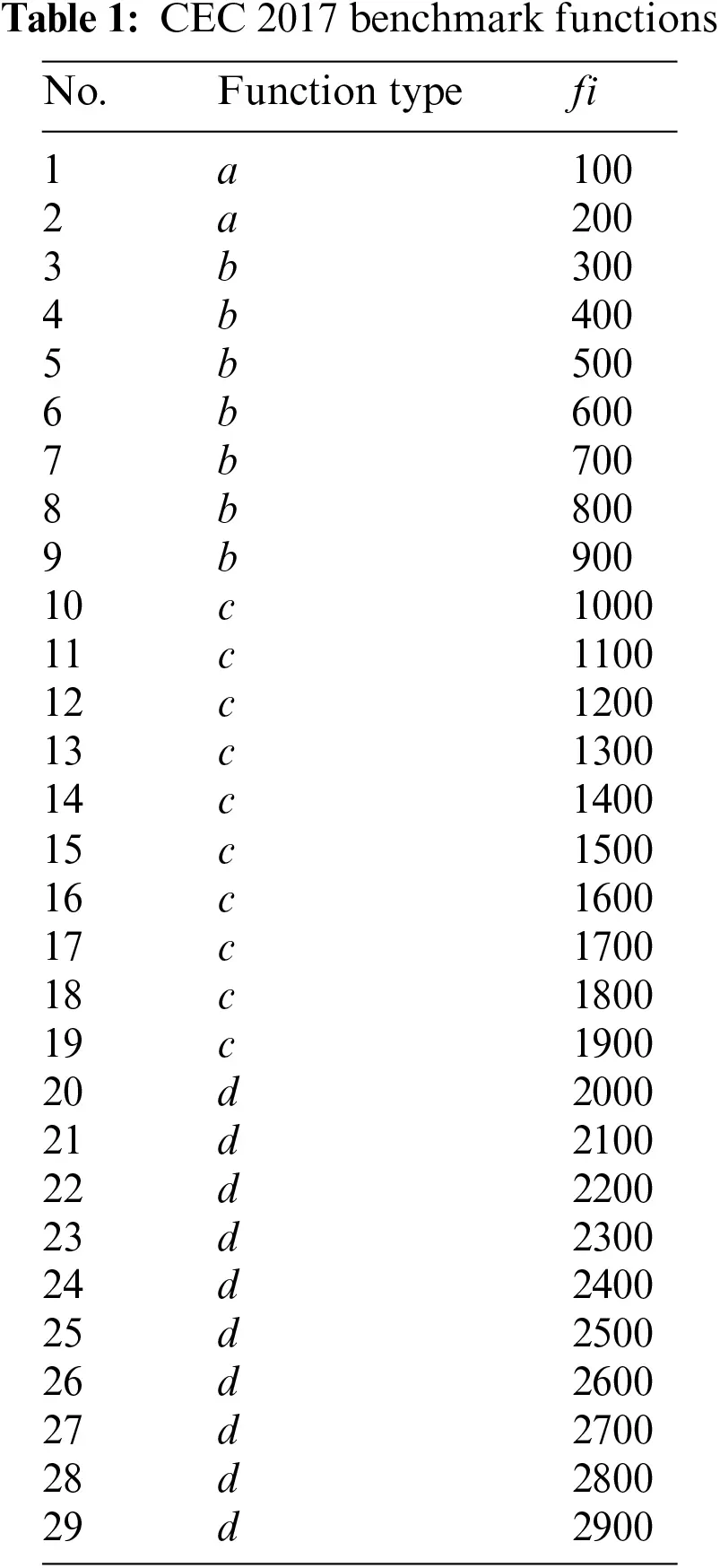The search range for all dimension variables in the function is [−100, 100]. All problems have global optima in a given range and do not change with dimension. The competition tests the optimization algorithm in four dimensions, namely 10D, 30D, 50D and 100D. The maximum number of iterations corresponding to each dimension is D * 104.

In addition, to eliminate the effects of randomness, each function is evaluated 30 times separately. Experimental findings are based on the deviation between the theoretical optimum and the actual value achieved by the algorithm. Five indexes are selected, namely ‘mean error (Me)’, ‘median error (Med)’,‘ Best error (Best)’, ‘Worst error (Worst)’, and ‘standard variance error (STD)’. All experiments are run on Windows 10 64 bit computer, using Intel i7 (3.2 GHz) processor and 8 GB RAM, and implemented in MATLAB R2018a environment.

4.2 Results and Discussion on CEC2017 Function set

The suggested CABES approach is compared to a number of other classical algorithms published in recent years in order to check its performance. They are PSO , WOA , AOA , BES  and YYFA .

This section studies 29 benchmark functions based on the CEC 2017 test set. All optimization methods are designed with the same experimental settings to assure the fairness and impartiality of the simulation trials, that is, the population numbers of all algorithms are equal, the loop stops when the maximum number of iterations is reached. The parameters in each algorithm take the values recommended in the original article, as stated in Table 2. Tables 36 provide results of the algorithm on 10D, 30D, 50D and 100D, respectively. Bold characters represent the least average error value and the best performance of the six algorithms. Figs. 710 show convergence curves of some function algorithms.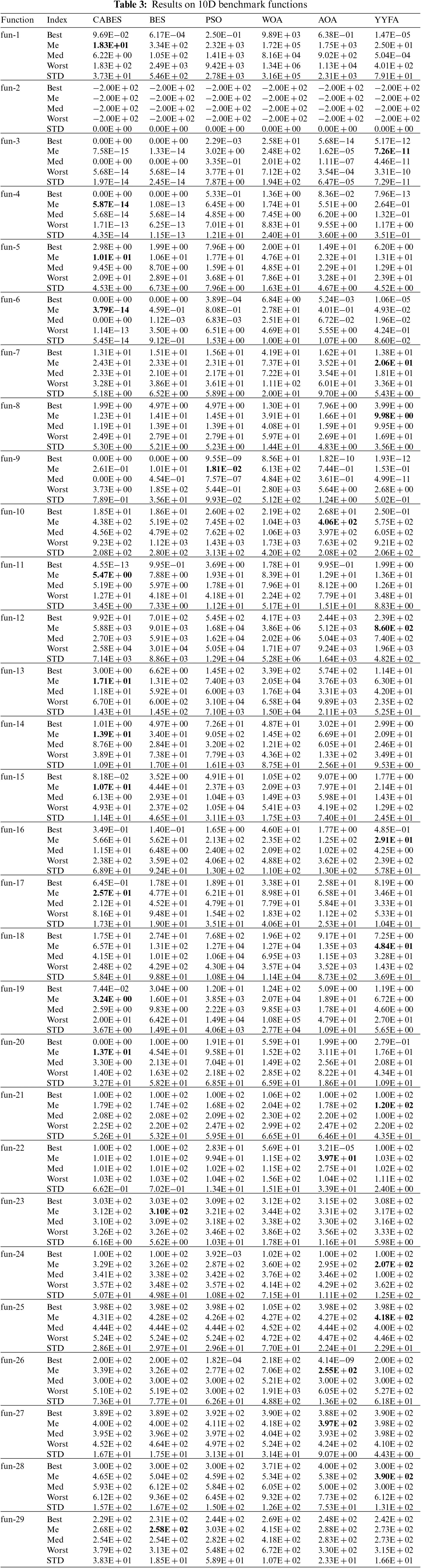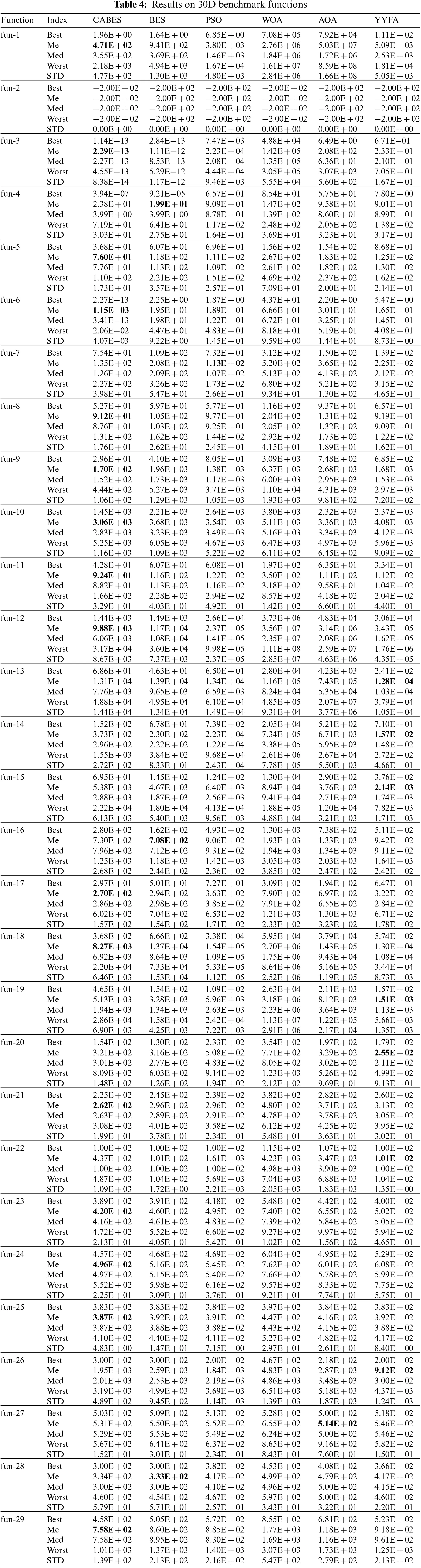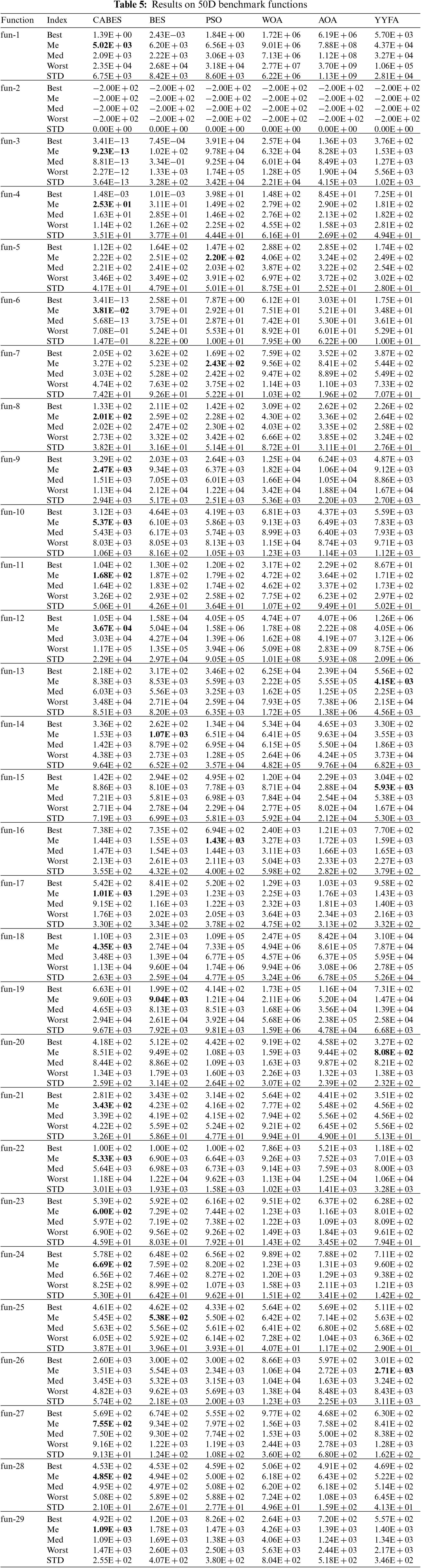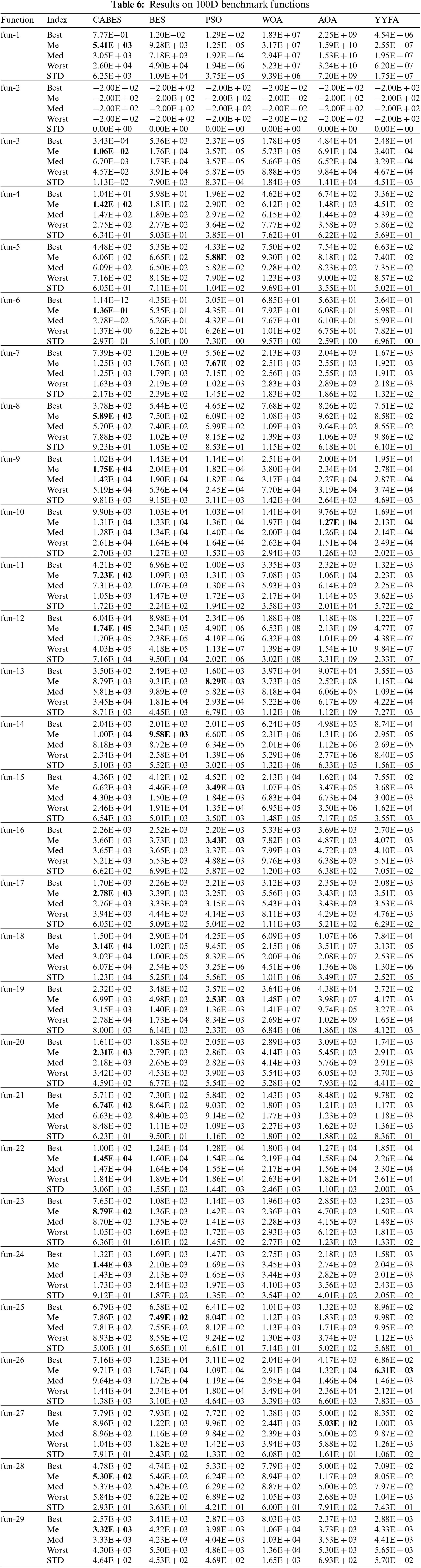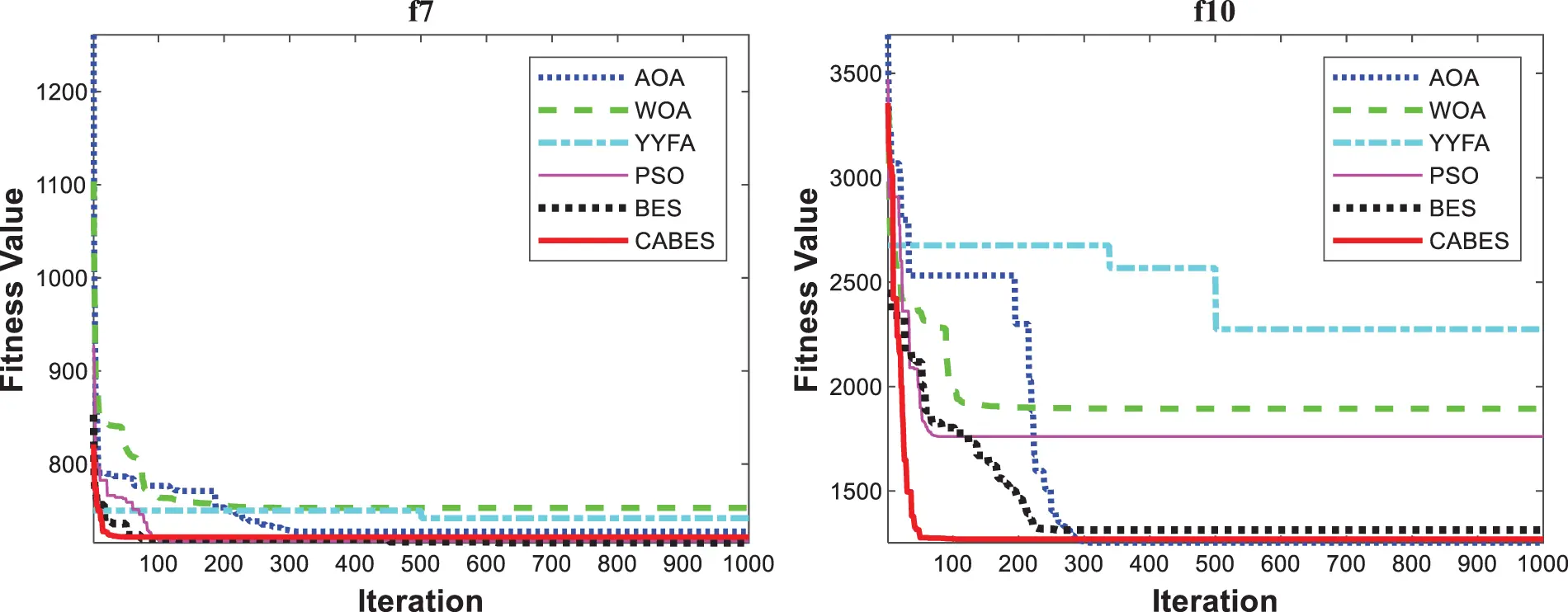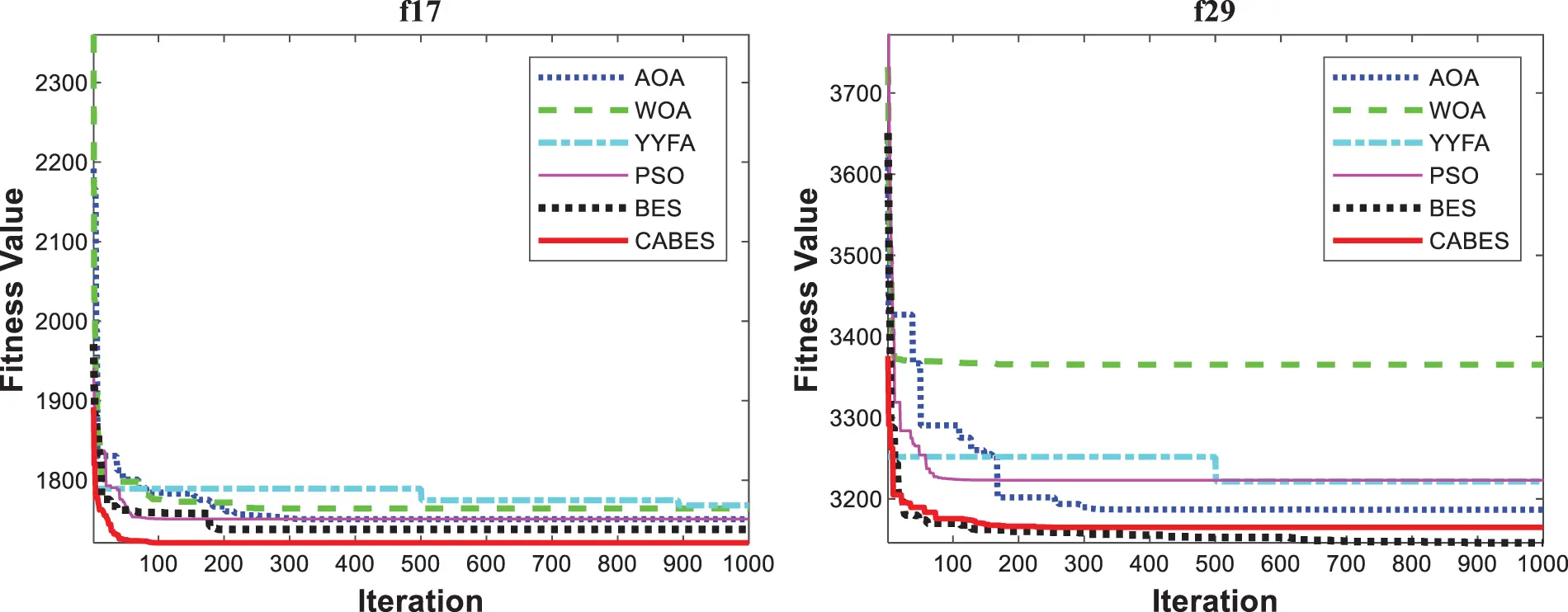Figure 7: 10D benchmark functions convergence curve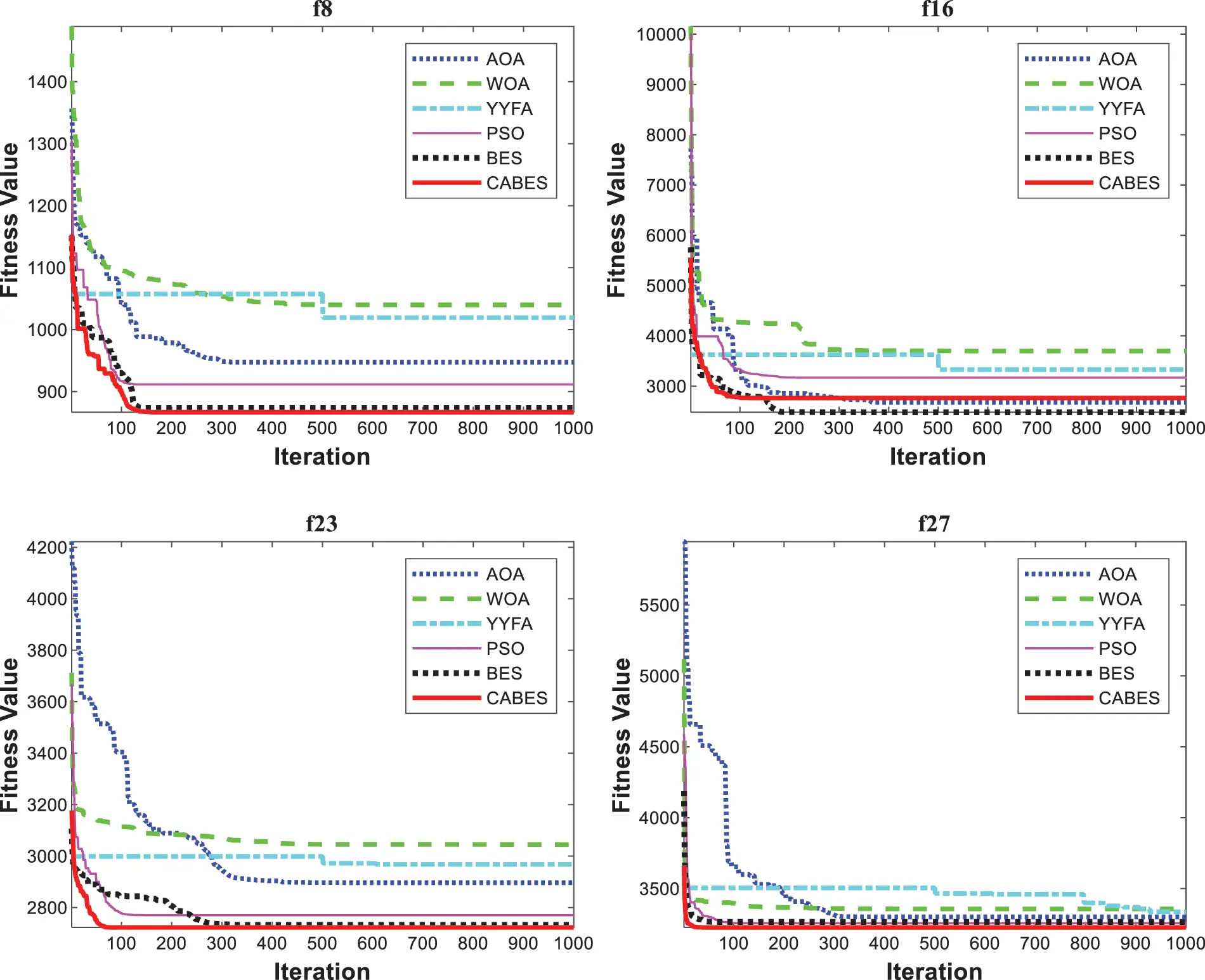Figure 8: 30D benchmark functions convergence curve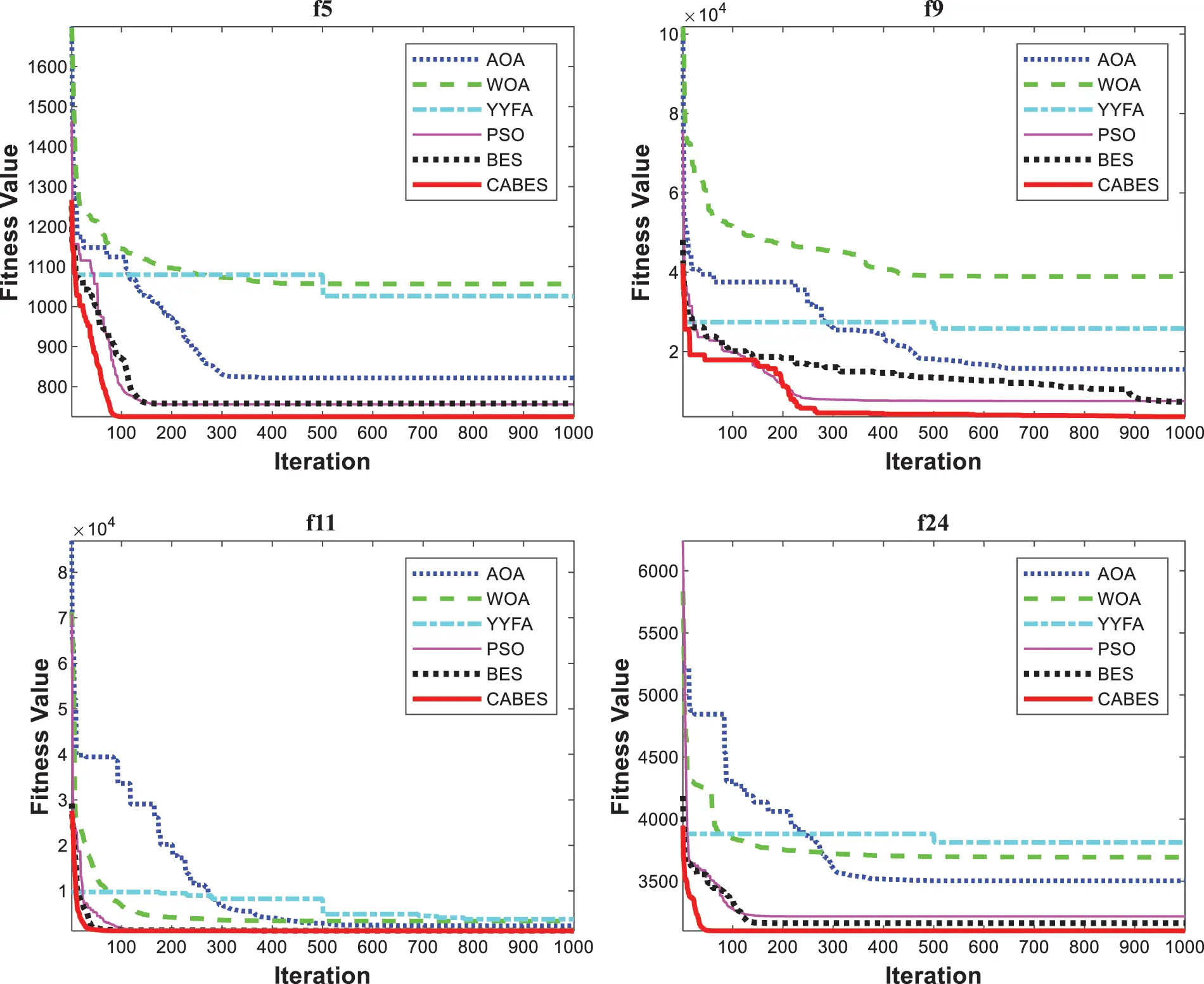Figure 9: 50D benchmark functions convergence curveFigure 10: 100D benchmark functions convergence curve

For the stability of the heuristic algorithm, we choose the percentage of the algorithm that is close to the optimal value in different execution processes to represent it. The higher the percentage, the greater the probability that the algorithm can reach the optimal value on the function, and the higher is the stability. The percentage error is selected as 30%, that is, the error between the actual value of the algorithm and the optimal value is within 30% of the optimal value, which will be considered as reaching a stable range. CABES and BES algorithms are selected for comparison. The algorithms are independently run 30 times on the CEC2017 test function set 10D and 50D, respectively. Due to the length of the article, the results are shown in Appendix of Table A1 and Table A2.

For 10D, CABES algorithm can reach the optimal value range in five functions, namely fun-1, fun-10, fun-12, fun-13 and fun-28, more than BES algorithm, and the other 23 functions can reach the optimal range. For 50D, only three functions, namely fun-1, fun-14, and fun-26, perform slightly worse than the BES algorithm, but perform better than the BES algorithm in 13 functions, and are equivalent to the BES in other functions. It can be considered that under different dimensions, through the test of multiple functions, CABES algorithm can obtain the optimal value better than BES algorithm and has a better stability. The results of the algorithm stability test are given in Appendix.

The results in Tables 36 indicate the following:

In the case of four different dimensions, CABES can produce more accurate results than the original BES algorithm in solving the functions fun-1, fun-3, fun-5-fun-13, fun-15, fun-17-fun-21, fun-24, fun-26, fun-27 in CEC2017. The complete optimization ability of CABES eventually outperforms that of BES as the dimension increases. This also demonstrates that CABES has a better ability to process data in high-dimensional situations.

According to the results from 10D to 100D in Tables 36,CABES is superior to other algorithms in 11, 16, 18 and 16 functions, respectively. For 100D, the PSO algorithm achieves better values than CABES on multimodal functions fun-5, fun-7 and hybrid functions fun-13, fun-15, fun-16 and fun-19. Therefore, compared with the PSO algorithm, CABES is more competitive in composition function. Compared with the AOA algorithm, CABES performs slightly worse on fun-27. Compared with the WOA algorithm, CABES performs better in different dimensions. On the whole, with the increase of dimensions, the optimization ability of CABES is improved more significantly than that of other optimization algorithms.

In case of 10D, the test value of CABES in functions fun-3, fun-7, fun-8, fun-12, fun-18, fun-21, fun-24, fun-25, and fun-28 is greater than that of YYFA. It shows that for 10D, the optimization ability of CABES in mixed function and composite function is inferior to that of YYFA. In the case of 30D, CABES still has some functions fun-13–fun-15, fun-19–fun-20, and fun-26 in terms of mixed functions and composite functions, whose optimization effect is not as good as that of YYFA. But on the simple multimodal functions fun-3, fun-7, fun-8, the mixed function and the composite functions fun-21, fun-24, fun-25, and fun-28 its performances are better than those of 10D, and the optimization ability is improved. In 50 and 100D cases, CABES is weaker than YYFA only in the optimization of fun-13, fun-15, fun-20, and fun-26 functions, while its performances are better than those of YYFA in other cases.

The experimental results of CABES in simple multimodal functions fun-3–fun-9 show that it has excellent exploration ability. The key reason is that CABES uses the Cauchy mutation approach (Eq. (15)), which increases the algorithm’s variety and improves the bald eagle’s global search capabilities. The experimental results of CABES on mixed functions and composite functions fun-10–fun-29 show that CABES can balance exploration and development, thus avoiding getting stuck in local optima. This is because CABES updates the search position update formula by introducing an adaptive weight factor (Eq. (16)), so that the update position is more accurate, and the local optimization ability of the bald eagle is improved.

The following are observed from Figs. 710:

a.   For functions of fun-1, fun-10, fun-11, fun-20, and fun-22 of CABES in 10D–100D, the curves drop vertically during the iterative convergence process, showing its ability to escape the local optimum.

b.   The convergence curves of multimodal, hybrid and composition functions fun-6, fun-8, fun-9, and fun-22 in 10D–100D show that CABES has a sharp continuous search ability within the specified number of iterations, indicating that the algorithm not only does not appear precocious, but also has the ability to continuously develop and excavate new solutions.

c.   According to the excellent performance of CABES on composition functions fun-20, fun-22, fun-23, fun-24, and fun-27 of 30D–100D, it demonstrates CABES’ ability to address complicated challenges.

d.   In the low-dimensional 10D case, CABES does not solve as accurately as BES on the composition function fun-29. However, CABES can achieve higher convergence accuracy with the increase of the dimension. It shows that CABES has a better ability to deal with high-dimensional problems.

In order to analyze the results quantitatively, a non-parametric statistical test, Friedman test, is used to evaluate the performance of each algorithm. The results are shown in Table 7. The smaller the rank mean is, the better the comprehensive performance of the algorithm is. It can be seen that the performance of CABES is slightly lower than that of YYFA only in the case of 10D, and the rank mean is the minimum in the case of 30D–100D, indicating that the performance of CABES is better than other algorithms with the increase of dimensions.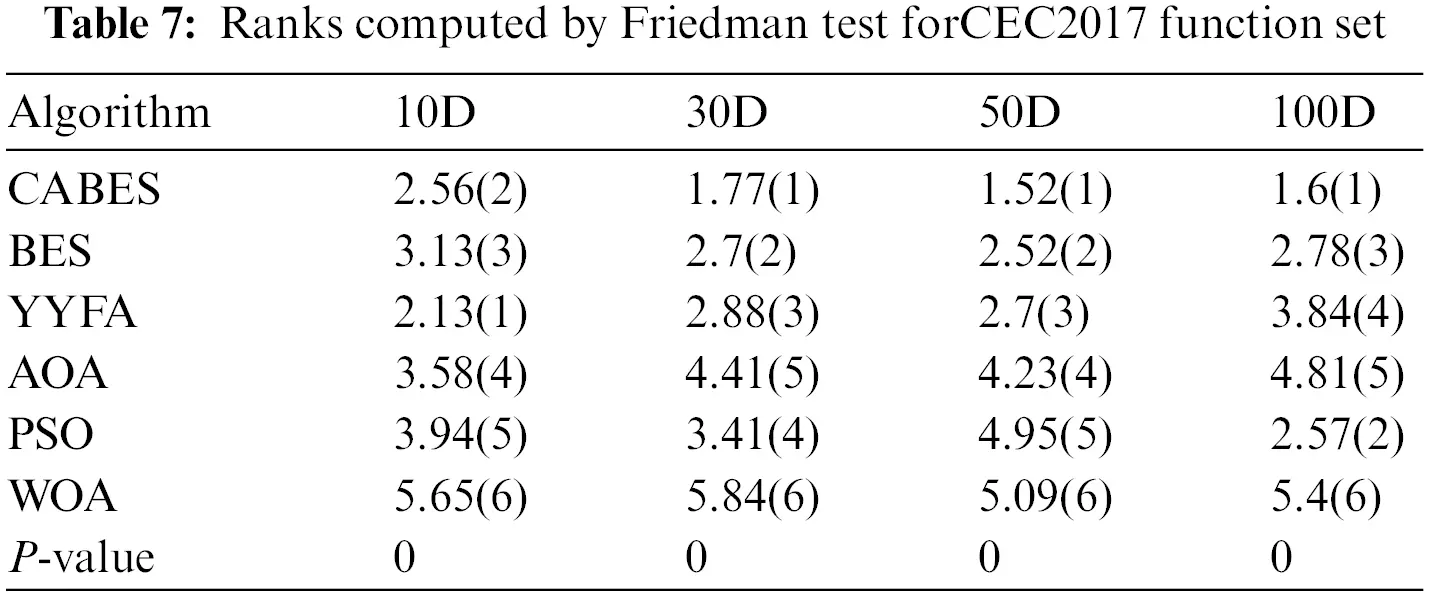In order to reflect the effectiveness of the improved algorithm, this paper uses Wilcoxon rank sum test to verify whether or not CABES is statistically significantly different from BES, PSO, WOA, AOA, and YYFA when the significance level P = 5% with different dimensions. The results are shown in Table 8. In the test functions, most of the P values are less than 5%. In general, the performance of CABES is statistically significantly different from other five algorithms, which shows that CABES has better effectiveness than other algorithms.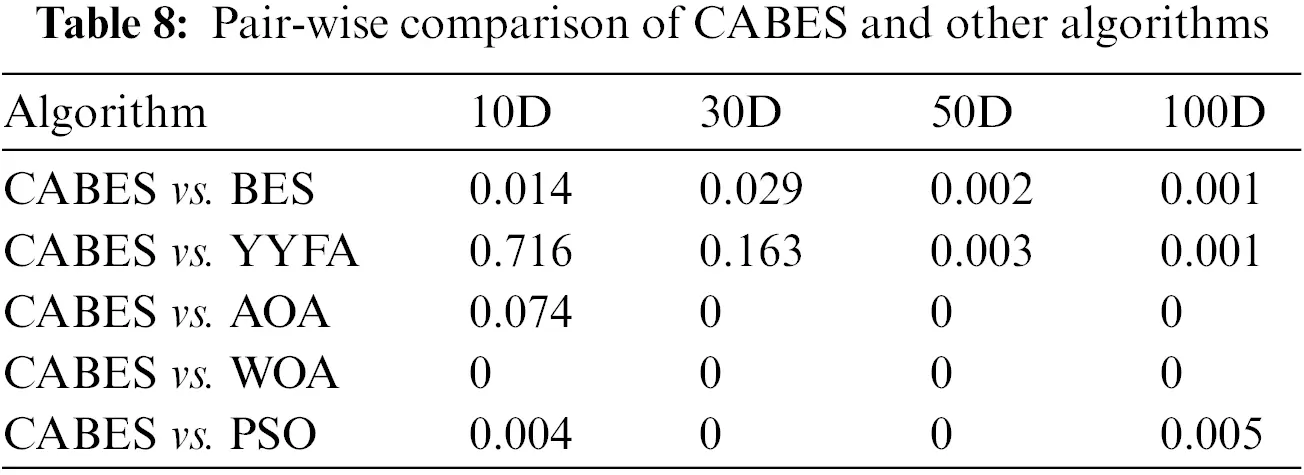4.3 Sensitivity Analysis of CABES Parameters

In Section 4.2, the performance of CABES and the original BES are tested, with results verifying the effectiveness of the improvement. Since the position change parameter is replaced by the Cauchy mutation operator in CABES, there are only three user-defined parameters, i.e., population number N, parameter s and controlling spiral trajectory t. Therefore, there is a need to constantly adjust the parameters in CABES to compare with BES. 29 benchmark functions in the CEC2017 test set are chosen for experiments under 30D conditions. The scheme and results of various combination parameters are shown in Table 9. Table 10 exhibits the non-parametric Friedman test ranking using mean error.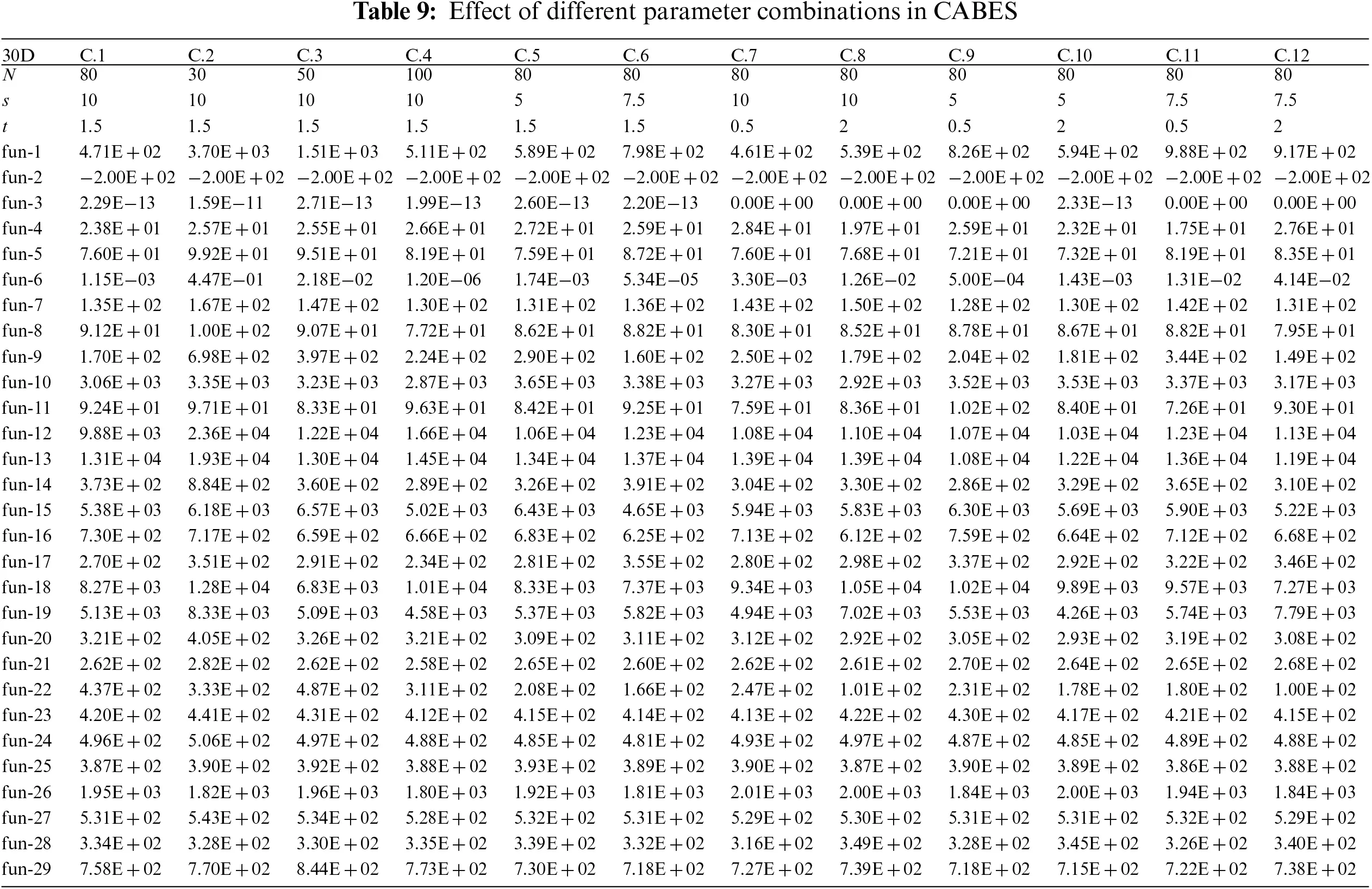The following are the effects of algorithm parameters, as shown in Tables 9 and 10:

a.   The effect of population number N: According to the previous four combinations, it can be found that the more the population number is, the better the optimization effect is.

b.   The position changing parameter s, which affects the angle of the eagle’s search and hunting: when the value is around 7.5–10, the optimization result is better, which increases the global search diversity. At around 5, the results show that it is not conducive to optimization convergence.

c.   Search period parameter t. According to the combinations seven and eight in Table 9, it can be observed that when t is about 0.5, it is conducive to convergence, but the changing of t alone has little effect on the algorithm. The joint effect of general and positional change parameter s is more obvious.

d.   To sum up, the parameter of population N has a great influence on the optimization effect of the algorithm. Ideally, the larger the population is, the better it is. The parameters s and t, which control the position change of bald eagles, have negligible impact on the overall optimization, and are not as obvious as the population size.

5  Performance in Practical Optimization Problems

5.1 Constrained Engineering Optimization Problems

To test the CABES algorithm’s performance in engineering optimization tasks, four standard constrained engineering problems are selected for performance evaluation. These four problems are speed reducer design (SRD), tension/compression spring (TCS), pressure vessel design (PVD) and welded beam design (WBD). The SRD challenge is to determine the reducer’s minimal weight. The TCS issue is a restricted problem with three variables and four constraints. PVD is a four-constraint optimization problem with four different types of variables. The WBD issue comprises five restrictions and four variables for the creation of welded beams.

The penalty function method (penalty factor 1030) is employed to deal with the above four constrained problems. Each problem is tested 50 times independently and compared to the BES algorithm’s initial version. The processing data is shown in Table 11. CABES can produce better solutions to four engineering challenges than the original BES, as shown in the table. It converges to the fitness value of 5885.332773616 for PVD, 1.695247165 for WBD, 0.01351617 for TCS and 2994.424465757 for SRD. Compared with BES, CABES obtains more reliable results, meets the constraints, and can address the constrained engineering problems better.5.2 Parameter Optimization in Groundwater Test Model

5.2.1 Groundwater Test Model

Pumping test is carried out for a well in a confined aquifer, and the water level drawdown of the observation well can be expressed by the analytical solution of the Tess model, the mathematical model of which can be found in references .

The fitness function adopted is shown in Eq. (17).

minf(T,S)=1Ni=1N(SSi)2s.tT[Tmin,Tmax],S[Smin,Smax](17)

where Si is the measured drawdown of water level at the i-th recording point, in m; N represents the total number of recording points of the pumping test.

5.2.2 Groundwater Experimental Simulation

In order to test the modified CABES algorithm’s dependability, a confined aquifer is used for the flow pumping test. The data and relevant parameters in the experiment are obtained from . It is known that the distance between the observation hole and the pumping well is r = 100 m. The main well is pumped with a constant flow, and the pumping capacity is q = 162.9 m3/min. The inversion parameters (T, s) are optimized by using CABES and other algorithms. The evaluation index includes root mean square error (RMSE), mean relative error (MRE), mean absolute error (MAE), with equations as Eqs. (18) to (20):

MRE=1ni=1n|s(i)s(i)/s(i)|×100%(18)

RMSE=1ni=1n(s(i)s(i))2(19)

MAE=1ni=1n|s(i)s(i)|(20)

where s(i) is the measured value; s(i) is the simulated value of the model.

The four algorithms are performed 30 times in a row. Please refer to Table 4 for test parameters. Table 12 shows the statistical findings.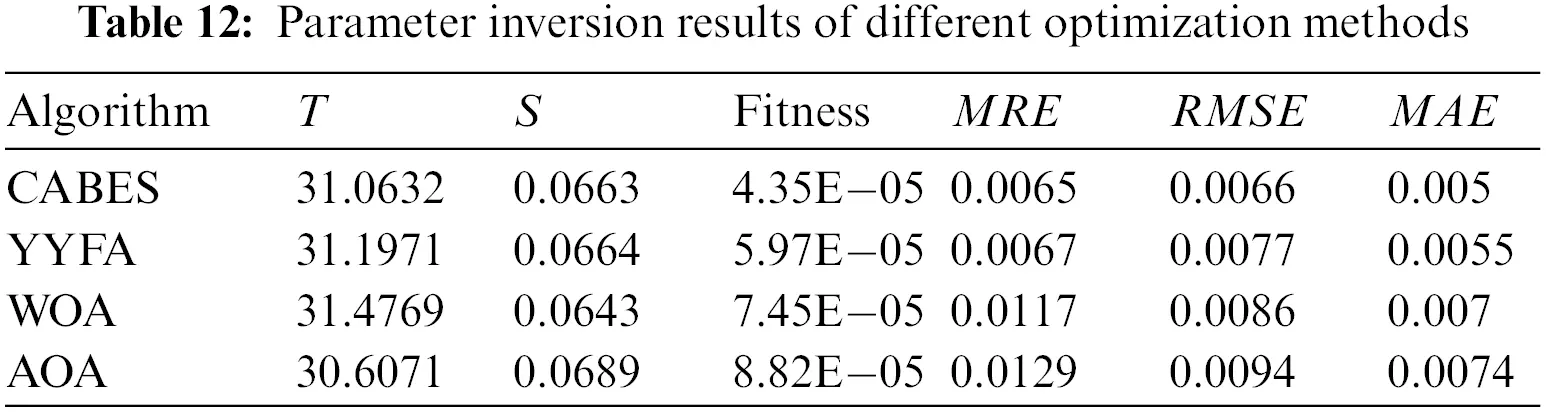5.2.3 Analysis of Results

(a)   The aquifer values obtained by the CABES algorithm are very close to those obtained by other methods. It is considered that CABES is an effective and feasible tool for parameter computation.

(b)   The CABES algorithm has the greatest inversion accuracy and fitness of 4.35E-05 when compared to other optimization algorithms, showing that the CABES algorithm has a more dependable global optimization capacity.

(c)   The order of the four algorithms in terms of error is as follows: CABES < YYFA < WOA < AOA.

(d)   The CABES algorithm ranks top because it has the minimum error index value. It is verified that the CABES algorithm has feasibility and competitiveness in the inversion of groundwater parameters.

6  Conclusions

This paper proposes an improved bald eagle search (CABES) based on the bald eagle search Algorithm (BES) for single objective optimization problems. The algorithm mainly combines the Cauchy mutation strategy and adaptive weight strategy, thereby strengthening the local mining ability of the original algorithm, improving the sufficiency of the vulture’s global search, effectively balancing the ability of local mining and global exploration, and avoiding the algorithm from falling into local optimization.

In the qualitative analysis of the algorithm, through comparison with other algorithms, 29 functions in the cec2017 test set are evaluated. The experimental results show that CABES performs better than other comparative algorithms in optimization ability and convergence accuracy when solving complex functions. While realizing strong development capability, it also ensures exploration performance, thus maintaining a good balance between development and exploration. Friedman test and Wilcoxon test also reflect the superior performance of the proposed algorithm in the statistical sense. However, according to the algorithm process, the time complexity of CABES is increased compared with that of BES, but they all belong to the same quantity set, which is acceptable.

Finally, the algorithm is applied to four different types of engineering design problems and a groundwater model, which further proves the applicability, effectiveness and superiority of CABES in optimization problems. It also shows the reliability of the CABES algorithm code proposed in this paper. Next, we will continue to improve the optimization mechanism of the bald eagle algorithm, improve its overall performance and to solve ability, apply it to more engineering design optimization problems, and further expand its application scope. In addition, we will also try to discretize the algorithm to solve discrete optimization problems.

Funding Statement: Project of Key Science and Technology of the Henan Province (No. 202102310259), Henan Province University Scientific and Technological Innovation Team (No. 18IRTSTHN009).

Conflicts of Interest: The authors declare that they have no conflicts of interest to report regarding the present study.

## References

1. Wang, W. C., Xu, L., Chau, K. W., Zhao, Y., & Xu, D. M. (2022). An orthogonal opposition-based-learning Yin–Yang-pair optimization algorithm for engineering optimization. Engineering with Computers, 38(2), 1149-1183. [Google Scholar] [CrossRef]
2. Mirjalili, S., & Lewis, A. (2016). The whale optimization algorithm. Advances in Engineering Software, 95, 51-67. [Google Scholar] [CrossRef]
3. Karaboga, D., & Basturk, B. (2007). A powerful and efficient algorithm for numerical function optimization: Artificial bee colony (ABC) algorithm. Journal of Global Optimization, 39(3), 459-471. [Google Scholar] [CrossRef]
4. Bansal, J. C., Sharma, H., Jadon, S. S., & Clerc, M. (2014). Spider monkey optimization algorithm for numerical optimization. Memetic Computing, 6(1), 31-47. [Google Scholar] [CrossRef]
5. Kennedy, J., Eberhart, R. (1995). Particle swarm optimization. Proceedings of ICNN. 95-International Conference on Neural Networks, vol. 4, pp. 1942–1948. Perth, Australia. DOI 10.1109/ICNN.1995.488968. [CrossRef]
6. Alsattar, H. A., Zaidan, A. A., & Zaidan, B. B. (2020). Novel meta-heuristic bald eagle search optimisation algorithm. Artificial Intelligence Review, 53(3), 2237-2264. [Google Scholar] [CrossRef]
7. Kirkpatrick, S., Gelatt Jr, C. D., & Vecchi, M. P. (1983). Optimization by simulated annealing. Science, 220(4598), 671-680. [Google Scholar] [CrossRef]
8. Hashim, F. A., Hussain, K., Houssein, E. H., Mabrouk, M. S., & Al-Atabany, W. (2021). Archimedes optimization algorithm: A new metaheuristic algorithm for solving optimization problems. Applied Intelligence, 51(3), 1531-1551. [Google Scholar] [CrossRef]
9. Mirjalili, S., Mirjalili, S. M., & Hatamlou, A. (2016). Multi-verse optimizer: A nature-inspired algorithm for global optimization. Neural Computing and Applications, 27(2), 495-513. [Google Scholar] [CrossRef]
10. Rashedi, E., Nezamabadi-Pour, H., & Saryazdi, S. (2009). GSA: A gravitational search algorithm. Information Sciences, 179(13), 2232-2248. [Google Scholar] [CrossRef]
11. Wang, L., Yang, R., Ni, H., Ye, W., & Fei, M. (2015). A human learning optimization algorithm and its application to multi-dimensional knapsack problems. Applied Soft Computing, 34, 736-743. [Google Scholar] [CrossRef]
12. Dai, C., Chen, W., Song, Y., & Zhu, Y. (2010). Seeker optimization algorithm: A novel stochastic search algorithm for global numerical optimization. Journal of Systems Engineering and Electronics, 21(2), 300-311. [Google Scholar] [CrossRef]
13. Holland, J. H. (1992). Genetic algorithms. Scientific American, 267(1), 66-73. [Google Scholar] [CrossRef]
14. Goh, C. K., Tan, K. C., Liu, D. S., & Chiam, S. C. (2010). A competitive and cooperative co-evolutionary approach to multi-objective particle swarm optimization algorithm design. European Journal of Operational Research, 202(1), 42-54. [Google Scholar] [CrossRef]
15. Zhang, Q., Sun, J., Tsang, E., & Ford, J. (2004). Hybrid estimation of distribution algorithm for global optimization. Engineering Computations, 21(1), 91-107. [Google Scholar] [CrossRef]
16. Ferahtia, S., Rezk, H., Abdelkareem, M. A., & Olabi, A. G. (2022). Optimal techno-economic energy management strategy for building’s microgrids based bald eagle search optimization algorithm. Applied Energy, 306, 118069. [Google Scholar] [CrossRef]
17. Zhou, J., Xu, Z., & Wang, S. (2022). A novel dual-scale ensemble learning paradigm with error correction for predicting daily ozone concentration based on multi-decomposition process and intelligent algorithm optimization, and its application in heavily polluted regions of China. Atmospheric Pollution Research, 13(2), 101306. [Google Scholar] [CrossRef]
18. Angayarkanni, S. A., Sivakumar, R., & Ramana Rao, Y. V. (2021). Hybrid grey wolf: Bald eagle search optimized support vector regression for traffic flow forecasting. Journal of Ambient Intelligence and Humanized Computing, 12(1), 1293-1304. [Google Scholar] [CrossRef]
19. Sayed, G. I., Soliman, M. M., & Hassanien, A. E. (2021). A novel melanoma prediction model for imbalanced data using optimized SqueezeNet by bald eagle search optimization. Computers in Biology and Medicine, 136, 104712. [Google Scholar] [CrossRef]
20. Kang, Z., Ren, F., Zhang, H., Lu, X., Li, Q. (2021). Diagnosis method of transformer winding fault based on bald eagle search optimizing support vector machines. 2021 IEEE 4th International Electrical and Energy Conference (CIEEC), pp. 1–5. Wuhan, China, IEEE.
21. Alabert, A., Berti, A., Caballero, R., & Ferrante, M. (2015). No-free-lunch theorems in the continuum. Theoretical Computer Science, 600, 98-106. [Google Scholar] [CrossRef]
22. Deng, L., Li, C., Han, R., Zhang, L., & Qiao, L. (2021). TPDE: A tri-population differential evolution based on zonal-constraint stepped division mechanism and multiple adaptive guided mutation strategies. Information Sciences, 575, 22-40. [Google Scholar] [CrossRef]
23. Rauf, H. T., Malik, S., Shoaib, U., Irfan, M. N., & Lali, M. I. (2020). Adaptive inertia weight Bat algorithm with sugeno-function fuzzy search. Applied Soft Computing, 90, 106159. [Google Scholar] [CrossRef]
24. Wang, Y., & Du, T. (2020). A multi-objective improved squirrel search algorithm based on decomposition with external population and adaptive weight vectors adjustment. Physica A: Statistical Mechanics and its Applications, 542, 123526. [Google Scholar] [CrossRef]
25. Liu, L., Zhao, D., Yu, F., Heidari, A. A., & Li, C. (2021). Ant colony optimization with Cauchy and greedy levy mutations for multilevel COVID 19 X-ray image segmentation. Computers in Biology and Medicine, 136, 104609. [Google Scholar] [CrossRef]
26. Chakraborty, F., Roy, P. K., & Nandi, D. (2019). Oppositional elephant herding optimization with dynamic Cauchy mutation for multilevel image thresholding. Evolutionary Intelligence, 12(3), 445-467. [Google Scholar] [CrossRef]
27. Jia, D., Wang, Z., & Liu, J. (2020). Research on flame location based on adaptive window and weight stereo matching algorithm. Multimedia Tools and Applications, 79(11), 7875-7887. [Google Scholar] [CrossRef]
28. Zhang, R., Yang, Z., Jiang, S., Yu, X., & Qi, E. (2022). An inverse planning simulated annealing algorithm with adaptive weight adjustment for LDR pancreatic brachytherapy. International Journal of Computer Assisted Radiology and Surgery, 17(3), 601-608. [Google Scholar] [CrossRef]
29. Liu, Q., Li, J., Wu, L., Wang, F., & Xiao, W. (2020). A novel bat algorithm with double mutation operators and its application to low-velocity impact localization problem. Engineering Applications of Artificial Intelligence, 90, 103505. [Google Scholar] [CrossRef]
30. Wang, W. C., Xu, L., Chau, K. W., & Xu, D. M. (2020). Yin-Yang firefly algorithm based on dimensionally Cauchy mutation. Expert Systems with Applications, 150, 113216. [Google Scholar] [CrossRef]
31. Wu, G., Mallipeddi, R., Suganthan, P. N. (2017). Problem definitions and evaluation criteria for the CEC 2017 competition on constrained real-parameter optimization. https://www.researchgate.net/publication/317228117_Problem_Definitions_and_Evaluation_Criteria_for_the_CEC_2017_Competition_and_Special_Session_on_Constrained_Single_Objective_Real-Parameter_Optimization.
32. Ali, M., & Pant, M. (2011). Improving the performance of differential evolution algorithm using Cauchy mutation. Soft Computing, 15(5), 991-1007. [Google Scholar] [CrossRef]
33. Gao, B., Shen, W., Zhao, H., Zhang, W., & Zheng, L. (2022). Reverse nonlinear sparrow search algorithm based on the penalty mechanism for multi-parameter identification model method of an electro-hydraulic servo system. Machines, 10(7), 561. [Google Scholar] [CrossRef]
34. Li, Y., Li, X., Liu, J., & Ruan, X. (2019). An improved bat algorithm based on lévy flights and adjustment factors. Symmetry, 11(7), 925. [Google Scholar] [CrossRef]
35. Li, Y., Zhao, Y., & Liu, J. (2021). Dynamic sine cosine algorithm for large-scale global optimization problems. Expert Systems with Applications, 177, 114950. [Google Scholar] [CrossRef]
36. Gan, C., Cao, W., Wu, M., & Chen, X. (2018). A new bat algorithm based on iterative local search and stochastic inertia weight. Expert Systems with Applications, 104, 202-212. [Google Scholar] [CrossRef]
37. Fan, Q., Huang, H., Chen, Q., Yao, L., & Yang, K. (2022). A modified self-adaptive marine predators algorithm: Framework and engineering applications. Engineering with Computers, 38(4), 3269-3294. [Google Scholar] [CrossRef]
38. Schramm, U., Möller, P., Tischer, A., & Reißmann, C. (1996). Adaptive mesh refinement using piecewise-linear shape functions based on the blending function method. Engineering with Computers, 12(2), 84-93. [Google Scholar] [CrossRef]
39. Srivastava, R. (1995). Implications of using approximate expressions for well function. Journal of Irrigation and Drainage Engineering, 121(6), 459-462. [Google Scholar] [CrossRef]
40. Singh, S. K. (2000). Simple method for confined-aquifer parameter estimation. Journal of Irrigation and Drainage Engineering, 126(6), 404-407. [Google Scholar] [CrossRef]
41. He, M., Zhou, J., Li, P., Yang, B., & Wang, H. (2023). Novel approach to predicting the spatial distribution of the hydraulic conductivity of a rock mass using convolutional neural networks. Quarterly Journal of Engineering Geology and Hydrogeology, 56(1), [Google Scholar] [CrossRef]

Appendix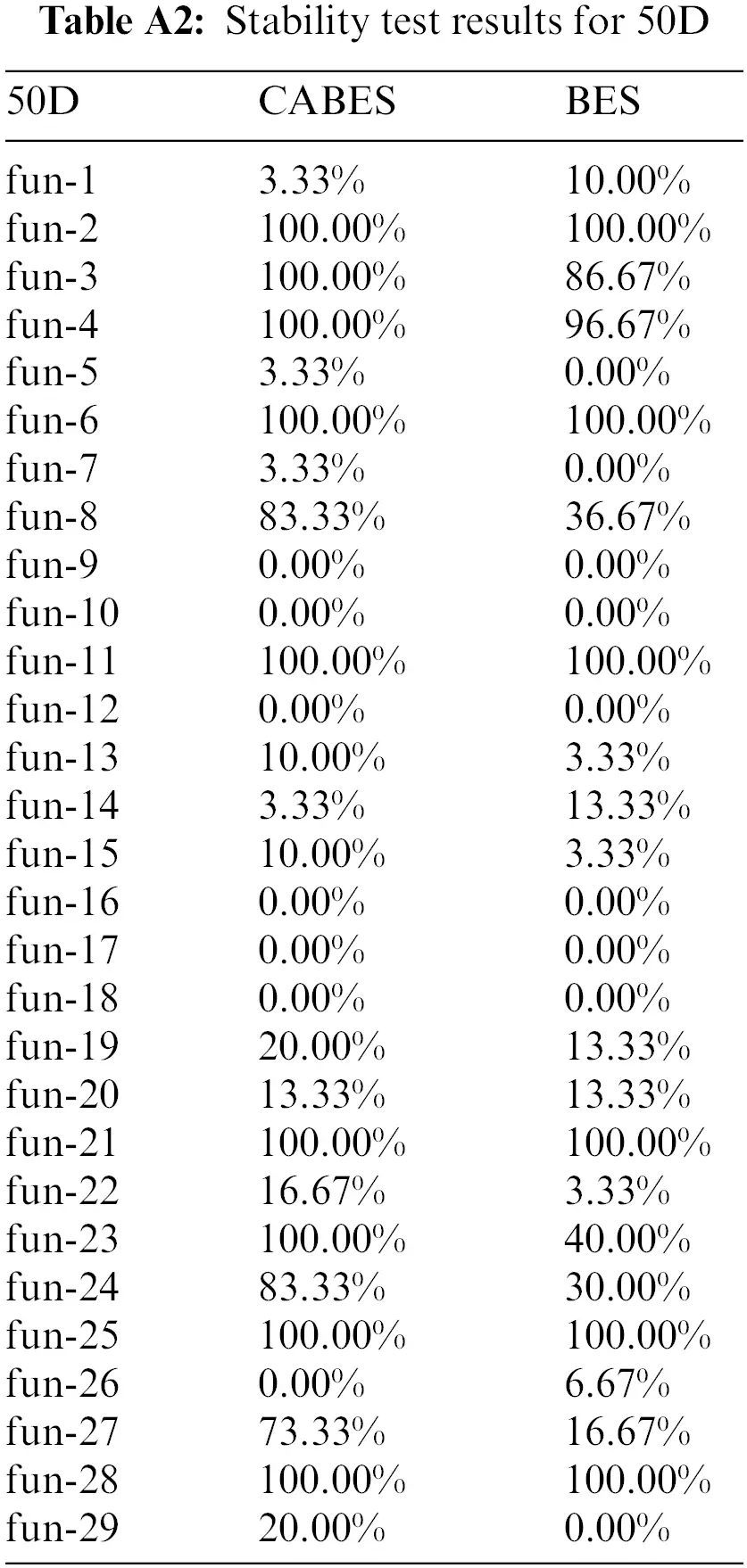Wang, W., Tian, W., Chau, K., Xue, Y., Xu, L. et al. (2023). An Improved Bald Eagle Search Algorithm with Cauchy Mutation and Adaptive Weight Factor for Engineering Optimization. CMES-Computer Modeling in Engineering & Sciences, 136(2), 1603–1642.This work is licensed under a Creative Commons Attribution 4.0 International License , which permits unrestricted use, distribution, and reproduction in any medium, provided the original work is properly cited.

View

Like

## Related articles

• M. Premkumar, R. Sowmya, Pradeep...
• Muhammad Asif Jan, Yasir Mahmood,...
• Hissah A. Ben Zayed, Mashael S....
• Huanwei Xu, Wenzhang Wei, Hanjin...
• Wenchuan Wang, Weican Tian, Kwok-wing...